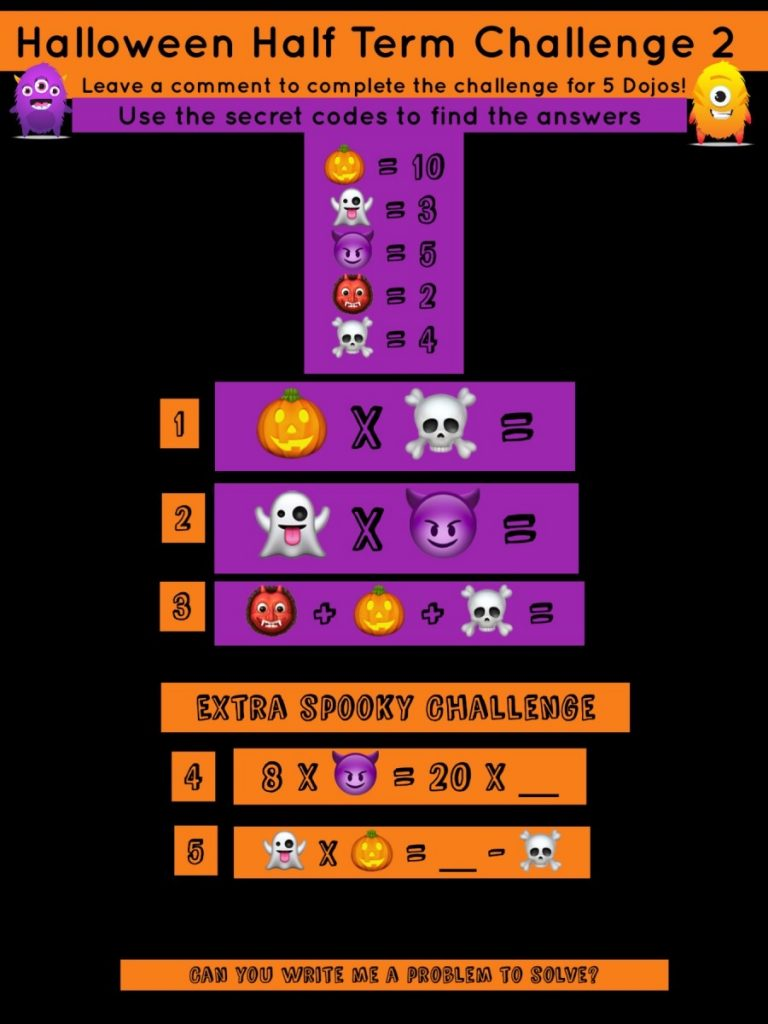### Halloween Half Term Challenge 2Chloe

1. 10 x 4 = 40
2. 3×5=15
3. 2+10+4=16
4. 8×5=20×2
5.3×10=34-4

Eben

1 = 40
2 = 15
3 = 16
4 = 20 x 2
5 = 34
Pumpkin x red devil = skeleton x – ?

Zara

1. 10×4=40,
2. 3×5=15,
3. 2+10+4=16,
4. 8×5=20×2,
5. 3×10=34-4

Ghost × skeleto4=_÷ vampire

Mayon

1. 30
2. 15
3. 16
PROBLEM MAKING: vampire, 5
zombie, 7
spider, 2
skeleton, 4

spider + zombie – 4 =
vampire x skeleton =
skeleton x 6 = 26 – 2 =
CHALLENGES: 4. 2
5. 34

1) 40
2) 15
3) 16
4) 2
5) 30

1. 40
2. 15
3. 16
4. 8 x 5 = 20 x 2
5. 3 x 10 = 34 – 4Mrs Lowrie

Teacher (MAT Leave)

I’m Mrs Lowrie, I teach year 2! I especially love key stage one and PE. I am pleased to say this is my eighth year of teaching at WCPS and I love to learn just like you! I enjoy spending time with my family and going for long walks.